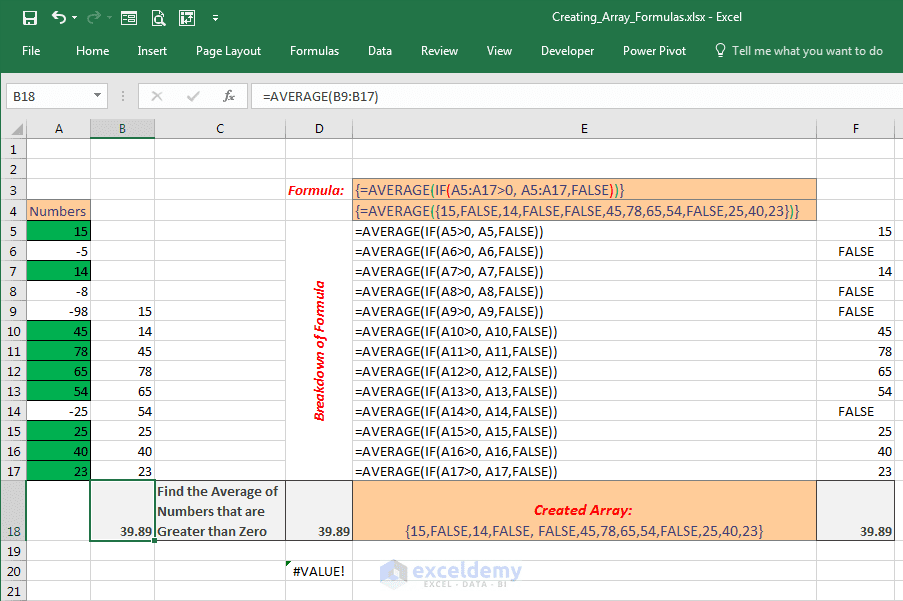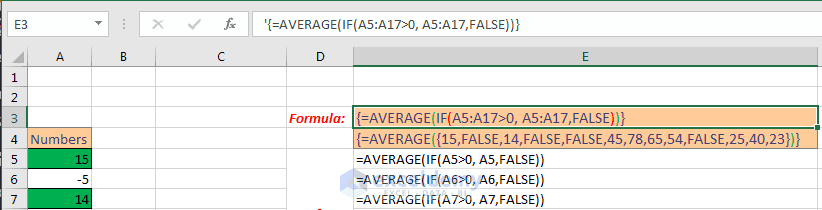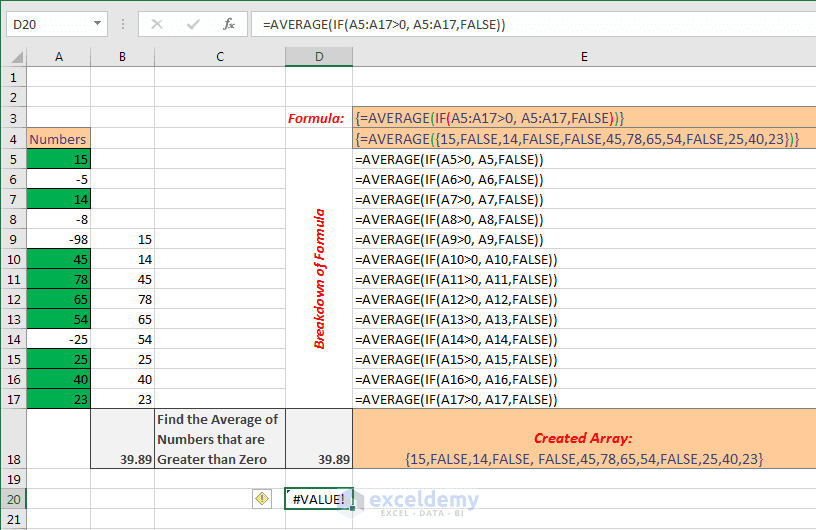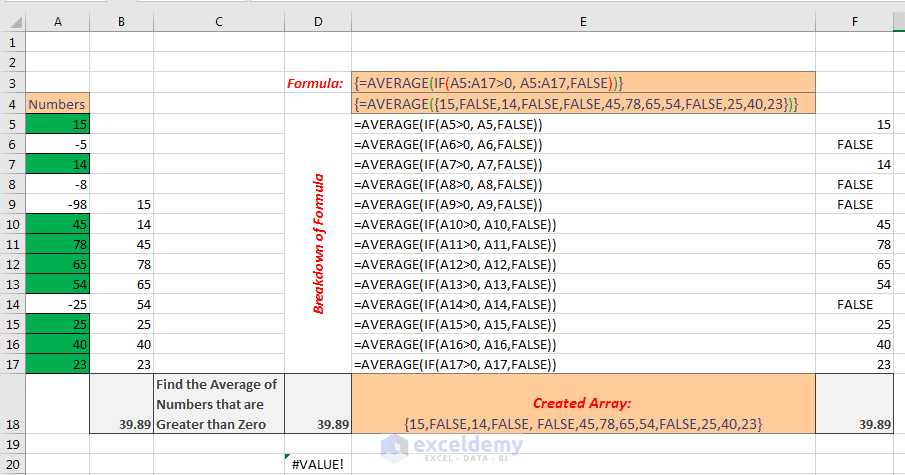# Excel Array Formula Basic 2 – Breakdown of Array Formula

If you’re a regular follower of my blog, you know that I have started writing a series of articles on Excel Array Formula. In the first article, I have demonstrated what are arrays in Excel and how to work (enter into a worksheet, edit) with them?

Read the first article here: Excel Array Formula Basic – What is an Array in Excel?

This is the second article of my series on Excel Array Formula. In this article, I will explain how an array formula works in the memory of Excel. “In the memory of Excel” means how Excel processes an array formula.

So, let’s start!

## Find out the average of positive numbers with an array formula

In this worksheet, you see there are some numbers on the left side of the worksheet.Excel Array Formula Basic [click on the image to get a clear view]

Some numbers are positive and some numbers are negative. I have highlighted the positive numbers with green fill and black border color. We are going to find out the average of the positive numbers of this range.

You can do it in two ways. The first way is: separate the positive numbers manually, create a new range (like I have done in column B), and at the end use the average function to find out the average of the positive numbers.

You see I have used the average function to find out the average of this separate range (Cell B18 is selected and look at the formula bar of the above image).

### Let’s do the same thing with an array formula

The other way is: we can use an Array Formula.

You can apply the array formula directly on the first range (from cell A5 to A17).

In cell D18, I have used the array formula and the result is showing same: 39.89.

To make you understand easily, I have copied the same formula in cell E3:

{=AVERAGE (IF (A5:A17>0, A5:A17, FALSE))}Closer look at the formula

You see the formula starts with the opening curly bracket, and closes with ending curly bracket, so this is undoubtedly an array formula.

You know this curly bracket is applied on an array formula automatically by Excel when you press CTRL SHIFT and ENTER keys simultaneously on your keyboard.

The next is an equal sign, this starts an Excel formula; then the average function, within the parenthesis of the average function, the green parenthesis is for the AVERAGE function, then IF function is placed. Red color parenthesis is for the IF Function.

Observe closely the arguments of the IF Function. You know how IF Function works. If the first argument of the IF function is TRUE, then the second argument is returned by the IF Function. If the first argument is FALSE, then the third argument is returned by the IF function. Arguments are separated by commas.

In our case, the third argument is FALSE, so if the first argument is FALSE, then the IF function will return the FALSE statement.

OKAY.

The main focus is now on the first argument and the second argument.

You see the first argument is a range, and the second argument is also a range. And the whole formula is an array formula.

You cannot deal this formula as a normal formula.

In the cell D20, I have entered this formula as a regular formula, and you see the cell is showing an error value (#VALUE! error).When you’re entering an array formula pressing Enter button, it is showing error.

The reason is IF function cannot work when you place a range as an argument. You are seeing two ranges are working as arguments in the IF Function. So it is showing the error.

We shall see now how Excel handles an array formula in step by step procedure.

Excel will break down these two ranges into single cells like shown in the following formulas.Observe the breakdown of the array formulas [click on the image to see a clear view].

The first breakdown is: find out the average if A5 is greater than 0, then A5 and then False, second breakdown is: find out the average if A6 is greater than 0, then A6 and then False, the third breakdown is: find out the average if A7 is greater than zero, A7 and then False and so on.

The first breakdown or the first formula returns 15, as the value of cell A5 is 15, and 15 is greater than zero, it is true; so the first formula returns A5 and A5 is 15. So the internally created array’s first value is 15. I am showing the internally created array in cell E3.

The second breakdown returns False, as the value of cell A6 is negative 5, so, A6 is not greater than zero, it is false, so it returns the third argument; the third argument is False. So the second value of the internally created array is False (see the array in cell E3).

The third breakdown formula returns 14 as A7 is 14, A7 is greater than zero, it is true, so it returns A7; A7 is 14. So the third value of the internally created array is 14.

So in this way, Excel creates this array internally: {15, FALSE, 14, FALSE, FALSE, 45, 78, 65, 54, FALSE, 25, 40, 23}

And the whole formula becomes like this one.

{=AVERAGE ({15, FALSE, 14, FALSE, FALSE, 45, 78, 65, 54, FALSE, 25, 40, 23})}

The IF function is totally replaced by the above Array. And the rest is simple. The AVERAGE function finds out the average of the values in the array, except the FALSE values, the False values are not numbers, so the AVERAGE Function neglects the FALSE values.

So this is how an array formula works. In this article, I have just introduced you with the Array Formulas.

In next some articles, I will deal with array formulas with more examples and in more details. Understanding array formula will reward you in many ways; you will be able to shorten a big regular formula into a small one and eventually becoming more professional in dealing with Excel formulas.

Array_Formulas_Breakdown.xlsx

## Summary

An Array Formula: {=AVERAGE(IF(A5:A10>0, A5:A10, FALSE))}

When you will enter the above formula into Excel as an Array formula, Excel will break down the formula in this way:

1. =AVERAGE (IF (A5>0, A5, FALSE))
2. =AVERAGE (IF (A6>0, A6, FALSE))
3. =AVERAGE (IF (A7>0, A7, FALSE))
4. =AVERAGE (IF (A8>0, A8, FALSE))
5. =AVERAGE (IF (A9>0, A9, FALSE))
6. =AVERAGE (IF (A10>0, A10, FALSE))

#### Kawser

Hello! Welcome to my Excel blog! It took me some time to be a fan of Excel. But now I am a die-hard fan of MS Excel. I learn new ways of doing things with Excel and share here. Not only how to guide on Excel, but you will get also topics on Finance, Statistics, Data Analysis, and BI. Stay tuned!

scott davis September 29, 2016 at 8:16 PM

Big-time Thank You!
I am finally starting to “get it”! So many texts and web helper sites seem to be speaking either to infants or to PHDs. Your tone and style is simple without being inane. Interesting without being unnecessarily complicated. Great job!

Kawser September 29, 2016 at 8:20 PM

Many thanks, Scott!

RAHUL November 5, 2016 at 12:50 PM

This is in awesome website and you are much more awesome Kawser!!!. I m loving excel now and will refer this to my friends too!!!. I will request you to plz share a practice worksheet containing advance excel problems for self practice. You should share the solutions on periodic basis. Thanks

Kawser November 6, 2016 at 11:13 AM

It’s a good idea, Rahul. I will think about it soon.
Best regards
Kawser

Jay April 27, 2018 at 11:32 PM

Really straight forward and easy to understand… I am off to search for more of these array posts from you. Excited to see which is next!

Jay April 27, 2018 at 11:46 PM

Searching for more array downloads seems challenging. Any advice on a course to take or docs to look for? I ultimately would like to make arrays that pull info from one tab to another based on a text selection in a row. Then based on that text selection – pulling all other rows that have a value and are not empty in that row to join it on another tab.

Excitied to try to build this array and do any course that teaches it!